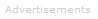# Definition of Significant Figures

Significant figures are used to indicate the precision of a number.For example, modern laboratory equipment in most cases can deliver more precise measurements than older equipment.

• An old voltmeter might have accurately recorded a voltage to be 1.2 V.
• A more modern one might accurately measure the same voltage to be 1.185 V.

Both of these results are accurate. However, the modern result is more precise, allowing it to confidently be quoted to four significant figures rather than the older result quoted to two significant figures.

Quick Guide to Writing Significant Figures
• The non-zero digits: 1,2,3,4,5,6,7,8,9 are all significant
• Zeros between non-zero digits are significant
• Zeros at the beginning of a number are never significant
• In numbers with a decimal point, zeros to the right of the last non-zero digit are significant
• In numbers without a decimal point, the significance of zeros to the right of the last non-zero digit is unclear - it should be clarified by providing further information or by writing as a decimal using exponents

### Significant Figures Examples

NumberSignificant Figures
122
1253
12544
125375
1.25375
1.253706
01.25375
1.2537007
1.2005377
12537005 or 7
1.2537 x 106 5
1.253700 x 106 7

In the table above, the number 1253700 has an ambiguous number of significant figures. Are the zeros truly in the measurement or are they merely placeholders? The number 1253700 is in the millions. Is it measured to a precision of one unit, or is it measured to the nearest 100?

The difficulty can be resolved as shown in the final two rows of the table by writing the number using exponents.Search the Dictionary for More Terms